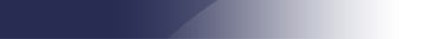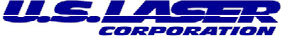825 Windham Court N.  Wyckoff, NJ 07481 (201)-848-9200## Laser solutions            for your world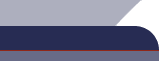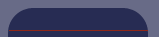News: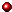Used LasersArticlesPress ReleasesNew ProductsTech. Notes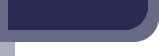Tech. Note

 [ Laser Shutters ] [ Measuring Laser Beam Divergence ] [ Beam Quality and Determination of M^2 ] [ Fiber Optic Beam Delivery Systems ] [ Fiber Optic Beam Delivery System Using Step Index Fiber ] [ High Power CW Nd:YAG Cavity Spacing Vs. Brightness ]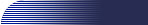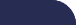Measuring Laser Beam Divergence Background: The National Institute of Standards and Technology, (NIST), has endorsed a laser beam divergence measurement technique that involves measuring the beam diameter at the ideal focal point of an imaging lens. A number of diameter measurement techniques can be used for focal plane divergence measurements including variable apertures, scanning pinholes, knife edges, and CCD arrays. U.S. Laser has adopted the CCD method for diameter measurements, in conjunction with the focal plane technique. The ideal focal point of a lens is defined as the point in space where the focus would be if the beam entering the lens was perfectly collimated. This point is also known in optics as the paraxial back focal point. This point is located exactly at a distance Fb from the vertex of the lens. Since all laser beams diverge, the actual focus is located farther from the lens than the ideal focal length (see Figures 1 and 2 for an illustration). For this application we are concerned only with the ideal focus and not with the actual focus. Once the beam diameter at the ideal focus is known, the beam divergence may be calculated using a simple formula. Selection of an Imaging Lens: The CCD imaging lens will have a back focal length of (Fb) millimeters, as measured from the vertex of the output surface. The CCD detector surface will be located exactly at the lens back focal length for the laser wavelength, regardless of whether there is actually a waist (focus) at that point. It is important that two conditions are met by the lens: 1. The working f-number, defined as the lens focal length divided by the beam diameter at the lens should be least 10, and preferably over 20. If the f-number is less than 10, aberration corrected optics must be used. 2. The lens should be selected such that the beam image on the CCD is as large as possible without overfilling. Overfilling the CCD array will result in false diameter readings. Underfilling the CCD will result in a loss of image resolution. A good rule of thumb is to have the CCD approximately ½ to ⅔ filled. Setup and Procedure: A schematic of the setup for divergence measurement is shown below in Figure 1. The procedure for measuring the divergence is as follows: 1. Set up the laser (or fiber), a lens of focal length F, and the CCD camera as shown in Figure 1. 2. Make sure the lens is oriented correctly (front-to-back) to minimize aberrations. The steepest curvature should face away from the CCD camera. 3. Place the CCD camera so that the pickup is exactly Fb millimeters from the center of the last surface of the imaging lens. 4. With the beam profiler, measure the beam diameter at this point in both the horizontal and vertical directions. Denote them as Dx and Dy. For these measurements use the diameters inside which 86.5% of the total power falls. 5. The divergences in the horizontal (x) and vertical (y) directions can be calculated using the formula: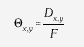where Dx,y is either Dx or Dy, depending on which axis is being measured, and F is the effective focal length of the lens at the laser wavelength. 6. For elliptical beams, the average divergence (average of x and y) can be calculated using the formula: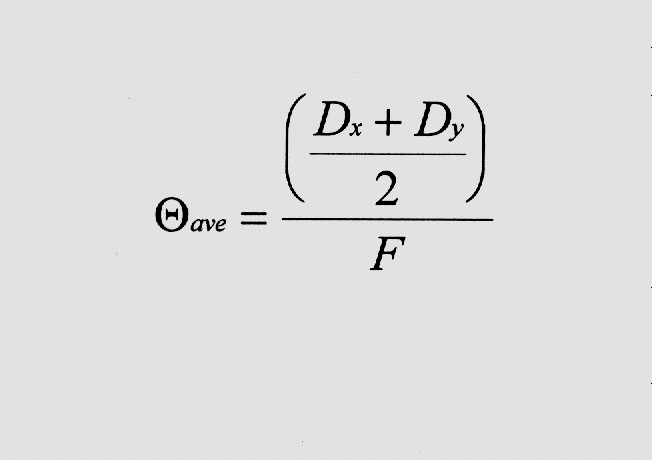References: 1. Laser Far-Field Beam Profile Measurements by the Focal Plane Technique, National Bureau of Standards, March 1978. 2. Simple Beam Propagation Measurements on Ion Lasers, SPIE Vol. 1414 Laser Beam Diagnostics, 1991. 3. Standard for the Measurement of Beam Widths, Beam Divergence, and Propagation Factors, Proposal for a Working Draft, ISO/TC 172/SC 9/WG 1, April 29, 1992.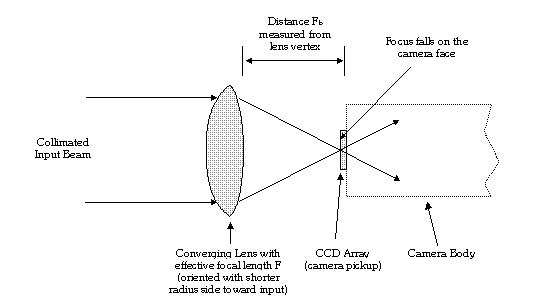Figure 1.  Setup for measuring divergence with lens and a CCD camera. The lens is placed a distance Fb from the lens vertex.  With a collimated input beam, the true focus falls directly on the CCD array.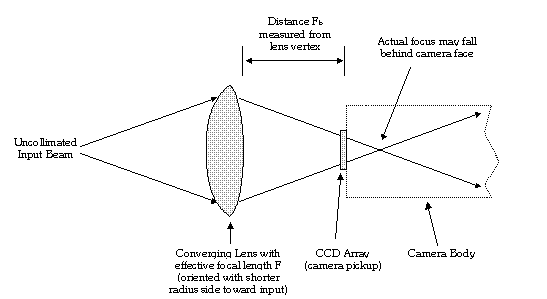Figure 2. Same setup as Figure 1, except with an uncollimated (diverging) input beam.  In this case, the true focus falls somewhere behind the CCD array.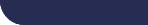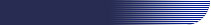Laser solutions for your world 825 Windham Court N. - Wyckoff, NJ 07481 Telephone: (201) 848-9200  -   Fax: (201) 848-9006  This page was last modified on: 4/11/2016